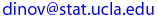# Due Date: Friday, Apr. 25, 2003, turn in after lecture

Correct solutions to any six problems carry full credit. See the HW submission rules. On the front page include the following header.

• (HW_2_1)  [Sec. 2.3,  #29]  The Student Engineers Council at a certain college has one student repre-sentative
from each of the five engineering majors (civil, electrical, indus-trial, materials, and mechanical). In how many ways can
(a) both a council president and a vice-president be selected?
(b) a president, vice-president, and a secretary be selected?
(c) two members be selected for the President’s Council?

• (HW_2_2)  [Sec. 2.3,  #33]  Shortly after being put into service, some buses manufactured by a certain company have developed cracks on the underside of the main frame. Suppose a particular city has 20 of these buses, and cracks have actually appeared in 8 of them.
(a) How many ways are there to select a sample of 5 buses from the 20 for a thorough inspection?
(b) In how many ways can a sample of 5 buses contain exactly 4 with visible cracks?
(c) If a sample of 5 buses is chosen at random, what is the probability that exactly 4 of the 5 will have visible cracks?
(d) If buses are selected as in part (c), what is the probability that at least 4 of those selected will have visible cracks?

• (HW_2_3) [Sec. 2.3,  #40]  Three molecules of type A, three of type B, three of type C, and three of type D are to be linked together to form a chain molecule. One such chain molecule is ABCDABCDABCDABCD, and another is BCDDAAABDBCC.
(a) How many such chain molecules are there?
(b) Suppose a chain molecule of the type desired is randomly selected. What is the probability that all three molecules of each type end up next to one another (such as BBBAAADDDCCC)?

• (HW_2_4) [Sec. 2.4,  #45] A certain sports car comes equipped with either an automatic or a manual transmission, and the car is available in one of four colors. Relevant probabilities for various combinations of transmission type and color are given in the table below. Let A = {automatic transmission} , B = { black } , and C = { white }.  Color White Blue Black Red Transmission Type A 0.15 0.10 0.10 0.10 M 0.15 0.05 0.15 0.20

(a) Calculate P(A), P(B), and P(A ∩ B).
(b) Calculate both P(A | B) and P(B | A), and explain in context what each of these probabilities represent.
(c) Calculate and interpret P(A | C) and P(A | C').

• (HW_2_5) [Sec. 2.5,  #72] Suppose that the proportions of blood phenotypes in a particular population are as follows
 A B AB O 0.42 0.10 0.04 0.44

Assuming that the phenotypes of two randomly selected individuals are independent of one another, what is the probability that both phenotypes are O? What is the probability that the phenotypes of two randomly selected individuals match?

• (HW_2_6) [Sec. 2.5,  #77] Two pumps connected in parallel fail independently of one another on any given day. The probability that only the older pump will fail is 0.10, and the probability that the newer pump will fail is 0.05. What is the probability that the pumping system will fail on any given day (which happens if both pumps fail)?

• (HW_2_7) [Sec. 2.5,  #75] An aircraft seam requires 25 rivets. The seam will have to be reworked if any of these rivets is defective. Suppose rivets are defective independently of one another, and each with the same probability.
(a) If 14% of all seams need reworking, what is the probability that a rivet is defective?
(b) How small should the probability of a defective rivet be to ensure that only 10% of all seams need reworking?

Last modified on by.

Ivo D. Dinov, Ph.D., Departments of Statistics and Neurology, UCLA School of Medicine